# Graphing Probability Distributions Associated with Random Variables

An error occurred trying to load this video.

Try refreshing the page, or contact customer support.

Coming up next: Finding & Interpreting the Expected Value of a Continuous Random Variable

### You're on a roll. Keep up the good work!

Replay
Your next lesson will play in 10 seconds
• 0:05 Random Events
• 0:49 Random Variables &…
• 2:25 Graphing a Discrete…
• 4:18 Graphing a Continuous…
• 5:09 Lesson Summary
Save Save

Want to watch this again later?

Timeline
Autoplay
Autoplay
Speed Speed

#### Recommended Lessons and Courses for You

Lesson Transcript
Instructor: Artem Cheprasov

Artem has a doctor of veterinary medicine degree.

What's a random variable? Does it have anything to do with gambling? What's the difference between a continuous and a discrete variable? This lesson explains the difference and how to graph each one.

## Random Events

Have you ever played the lottery or tried your luck at the casino? If you're not old enough, then maybe you know of someone who has. A lot of events and processes in the world, including the ones you might find at a casino, have a random nature to them.

Some examples of random processes include drawing lottery numbers, playing the roulette wheel, and even measuring the yearly rainfall. All of these can be quantified with random variables and something called the probability distribution function. In this lesson, you will learn how to graph probability distributions that result from random processes.

Why don't we start by defining terms like random variable and probability distribution function before taking a look at some examples related to these concepts?

## Random Variables and Probability Distribution

A random variable is a quantity that designates the possible outcomes of a random process. It's used to map the potential outcomes of a random process to numeric values. Random variables can be associated with both discrete and continuous processes. Processes that can be described by a discrete random variable include flipping a coin, picking a number at random, and rolling a die.

Conversely, examples of events associated with a continuous random variable include the height and weight distribution of people within a population. A good way to determine if the random variable is discrete or continuous is as follows: if there is a countable number of values that the random variable can take on, then it is discrete; otherwise, it is continuous.

In the intro, I also mentioned something called the probability distribution function. The probability distribution function is a function that describes the likelihood of all the possible values that the random variable can take on.

If the random variable is discrete, then the corresponding probability distribution function will also be discrete. Alternatively, if the random variable is continuous, then the associated probability distribution function will also be continuous. Easy, right?

One thing, though. It's important to note that in the case of continuous random variables, the probability distribution function is also called the probability density function. Let's take a look at how to graph these probability distributions.

## Graphing a Discrete Probability Distribution

Let's first turn our attention to graphing a discrete probability distribution. When rolling a six-sided die, the random variable can have the values 1 through 6, as shown on the screen: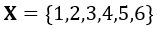If the die is fair, meaning that there is no preference for any particular outcome, then the probability of rolling any number (say, a 5) is the same as the probability of rolling any other number (say, a 3).

Since there are only six possible values the random variable can take on, it is discrete. The probability distribution function, also being discrete, would show the probability of rolling any integer number between 1 and 6, inclusive. Mathematically, this function can be written as follows: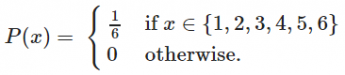Graphically, the probability distribution function is represented by a bar graph, with the x-axis denoting the values that the random variable can take on and the y-axis denoting the probability of each outcome. The probability distribution graph is shown for you on-screen for our example.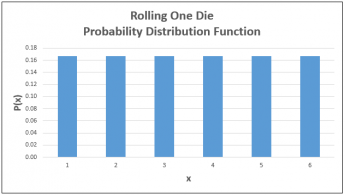Okay, let's take a look at another example. We can define a random variable, x, associated with rolling two six-sided dice, as follows: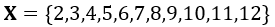The random variable can take on the shown values because the lowest possible outcome is rolling a 1 on both dice, while the highest possible outcome is rolling a 6 on both dice, hence the 12. The probability distribution function, then, would be shown as follows: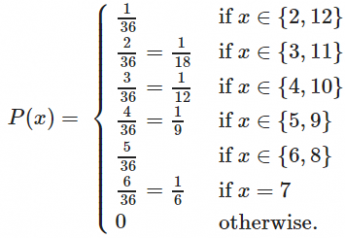To unlock this lesson you must be a Study.com Member.

### Register to view this lesson

Are you a student or a teacher?

#### See for yourself why 30 million people use Study.com

##### Become a Study.com member and start learning now.
Back
What teachers are saying about Study.com

### Earning College Credit

Did you know… We have over 200 college courses that prepare you to earn credit by exam that is accepted by over 1,500 colleges and universities. You can test out of the first two years of college and save thousands off your degree. Anyone can earn credit-by-exam regardless of age or education level.